Next: The Effect of Relativistic Up: Ejectors in Massive Binary Previous: Why do Radiopulsars not

## Caverns in Binary Systems

Ā

We follow the approach used by Lipunov and Prokhorov (1984). Suppose that a NS having a rotational magneticĀ luminosityforms a binary system (with semi-major axis a) with a normal star losing matter in the form of stellar wind (with a loss rate).

Let us find the Schwartzman radiusĀ in accordance with the definition and by equating the plasma pressureto the electromagnetic pressure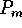:where R is the distance from the NS. The Schwartzman radiusĀ is (see Lipunov and Prokhorov, 1984; Harding, 1991):When making estimates, it is convenient to use the fact that the outflow rate, luminosity and the stellar wind velocity of hot stars are connected through the empirical relation (see, for example, Barlow and Cohen, 1977)where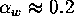-0.4. This leads to the approximation

ĀThe luminosity of normal stars whose mass varies from a few times to several tens of times the mass of the Sun lies in the interval between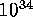and-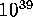erg s. The rotational magnetic luminosityĀ of NS also varies in the same wide range. For ``aged'' radiopulsars,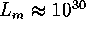erg s, while for young pulsarsmay be as high as-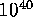erg s. Equation (10.4.3) shows that entirely different situations may arise in different binary systems at various stages of evolution. For example,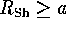or. This means that while deriving the formula for a cavern in the general case, we must take into account the proximity of the normal star.

Ā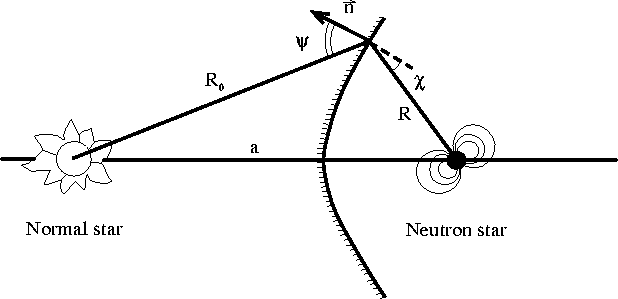Figure 28: Schematic representation of a pulsar in a pair with a normal star.Ā

Let us calculate the shape of a cavernĀ surrounding a NS, assuming that the relativistic wind ejected by the star is spherically symmetric. We also assume that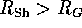. At the cavern boundary (Figure 28), the following equilibrium condition must be satisfied:

Ā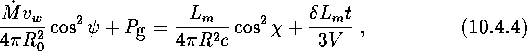whereis the distance from the normal star, V is the volume of the cavern, andis the gas pressure. The second term on the right-hand side of the equilibrium equation (10.4.4) corresponds to the contribution of the pressure of the magnetic dipole radiation which is reflected back by the cavern ``walls'' and is accumulated inside it. The factor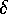is zero for an open cavern and approximately 1 for a closed cavern. The static gas pressure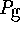can be presented in the form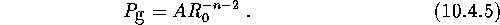For an isothermal stellar windĀ (as a rule, this is the situation commonly encountered), n=0. We use dimensionless variables:In this case, equation (10.4.4) becomesLet us consider the case of an isothermal stellar wind (n=0) with no magnetic dipole radiation accumulated (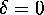):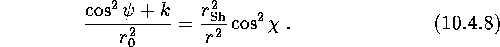It follows from the symmetry considerations that at the anterior point of the cavern (i.e. the point closest to the normal star),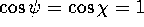. This leads to the following expression for the distance from the anterior point: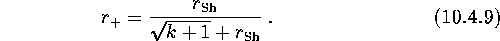Similarly, we can determine the distance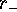from the posterior point of the cavern. We getIf, the cavern is closed. The value of k is of the order of the ratio of the square of the velocity of sound to the outflow rate of the stellar wind, so that in real situations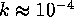-. Hence closed caverns must be quite compact:-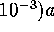. In most cases, the assumption k=0 may serve as a good approximation.

Only the electromagnetic waves with a frequency higher than the plasma frequency (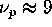Hz) may propagate in a plasma. The characteristic density in the stellar wind is estimated from the continuity conditionwhere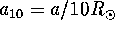. Thus, the magnetic dipole radiationĀ should be reflected from the walls. Suppose that the reflection coefficient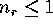. In this case, the above analysis will remain valid if we redefine the Schwartzman radius as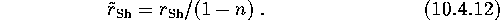This givesIt can be seen that the size of the cavern has increased. As, the cavern cannot remain stationary. It is either always open or pulsating quasi-periodically. In the latter case, the closed cavern expands right up to the maximum value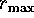: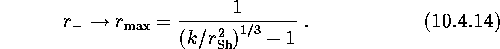The shape of the closed cavern is shown in Figure 29.

ĀFigure 29: The cavern around a pulsar in a binary system (Lipunov and Prokhorov, 1984).ĀĀNext: The Effect of Relativistic Up: Ejectors in Massive Binary Previous: Why do Radiopulsars not

Mike E. Prokhorov
Sat Feb 22 18:38:13 MSK 1997